Navigation C API Pages Python bindings Applications

## Templating Engine

The GFXprim uses python templating engine to generate code for different pixel types. This is documentation to the engine internals.

### GFXprim Config

The configuration which describes pixel layout could be found in root GFXprim directory under name gfxprim_config.py which is used to create objects described below.

### Main Objects

#### Pixel Size

```class PixelSize(object):
self.size = size

self.suffix = suffix

self.bit_endian = bit_endian

def needs_bit_endian(self):
...

...
```

The Pixel Size object represents pixel as a continous block of data.

The size is size in bits and is sometimes called bpp which stands for "bits per pixel". This number includes padding bits, if any.

The suffix is a string used as a suffix for functions, macros and other identifiers that operate with this pixel size.

The bit_endian determines the ordering of pixel bits within a byte in bitmaps and graymaps. This is significant in pixel types with pixel boundaries within a byte, i.e. 1 bpp, 4 bpp or 17 bpp.

The function needs_bit_endian() returns true for pixel sizes that are not aligned to the whole bytes.

The bit endian can be either BE or LE (i.e. most significant bit left or right in the bitmap). Usually, the bitmap is ordered with left bits earlier in the memory, but the earlier/later relationship is important. This is defined in terms of natural pixel ordering within the memory.

BE means that the earlier (usually left-most) pixels use the higher ("big") bits of the byte. I.e. in 1 bpp, the first pixel would have value 128, the second 64 and so on.

LE means that the earlier (usually left-most) pixels use the lower ("little") bits of the byte. I.e. in 1 bpp, the first pixel would have value 1, the second 2, the eight’th 128 and so on.

The following two figures describe bit layout in 1 bpp and 2 bpp grayscale bitmaps. The rectangles in the figures represents bytes as they are in the bitmap memory. The start of the image (i.e. topleft corner and coodinates (0,0)) is on the left side. The numbers describe position of the bit in the byte, i representing value 1<<i, that is 7-th bit representing value 128 and 0-th bit value 1.

##### BE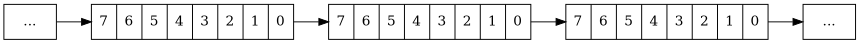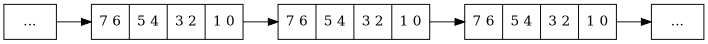##### LE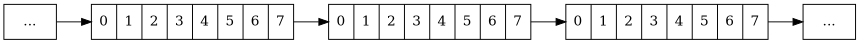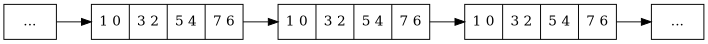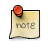Different pixel types (i.e. RGB888 and BGR888) can share the same pixel size, since certain types of operations do not depend on the actual arrangement of the color channels in a pixel (i.e. get/put pixel, rotate buffer 90 degrees, etc.).

TODO: Rename size to bpp?

#### Pixel Type

```class PixelType(object):
self.name = name
self.chanslist = chanslist
self.chans = dict() # { chan_name: (offset, size) }
self.pixelsize = pixelsize # bits per pixel

def is_palette(self):
...

def is_unknown(self):
...

def is_rgb(self):
...

def is_cmyk(self):
...

def is_gray(self):
...

def is_alpha(self):
...
```

This object represents pixel type which describes the sizes and arrangements of channels in a pixel. Note that it carries an instance of pixel size described above.

```class PixelChannel():
# Index (position in pixel from left)
self.idx = idx
# Pixel channel name such as R, G, B, A, ...
self.name = name
# Bit offset in pixel
self.off = offset
# Channel bit size
self.size = size
# Maximal channel value
self.max = 2 ** size - 1
...```

The chanslist describes pixel channel. There are some convenient members to be used directly from C code whose value is a hexadecimal string, i.e. C_mask, C_max, C_shift.

### Templating language

GFXprim uses CCT templating engine which is crafted especially for generating source code. CCT is a Python based language that contains global variables with objects described in gfxprim config.

#### Quick Syntax Help

CCT basics:

• Each written line is verbatim (reproduced as it is) unless it starts with `@\s`

• Lines starting with `@\s` are interpreted as a Python code

• Comments are lines starting with `@ #`

• Verbatim lines may also contain Expressions or Function call

• Expression is a Python code that yields a value which is included in the output and is enclosed between curly braces `{{ expression }}`.

• Function call is a Python function whose output is included in the output. It is called as {@ func() @}.

More complete documentation could be found in CCT howto.